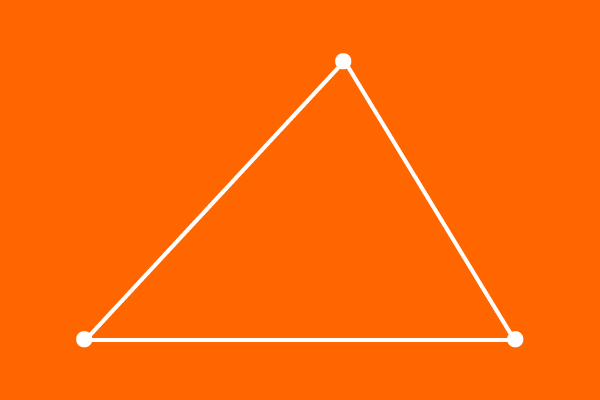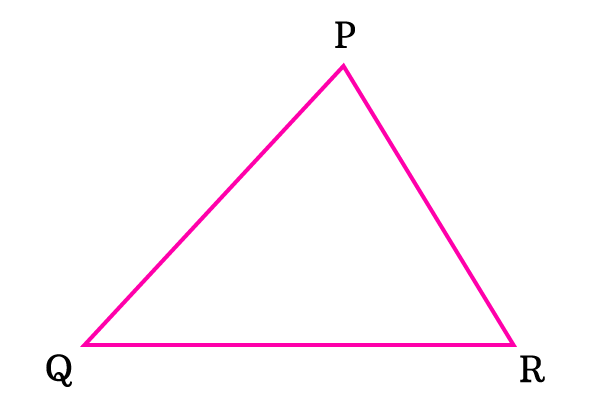# Vertices of the triangle## Definition

A meeting point of every two of three sides of the triangle is called a vertex of the triangle.

Three line segments form a triangle geometrically by the meeting of every two line segments at their endpoints. So, total three meeting points are formed in every triangle.

Each meeting point of every two sides in a triangle is called vertex of the triangle and all three meeting points are called vertices of the triangle.

### ExampleTriangle $PQR$ is formed by three line segments. The three line segments meet mutually at three different points $P$, $Q$ and $R$ to form this triangle.

These three meeting points of the sides are vertices of the triangle $PQR$.

#### First vertex

The sides $\overline{PQ}$ and $\overline{PR}$ meet at the point $P$. So, the point $P$ is vertex of the triangle. The point $P$ is called as vertex $P$.

#### Second vertex

The sides $\overline{PQ}$ and $\overline{QR}$ meet at the point $Q$. So, the point $Q$ is vertex of the triangle. The point $Q$ is called as vertex $Q$.

#### Third vertex

The sides $\overline{QR}$ and $\overline{PR}$ meet at the point $R$. So, the point $R$ is vertex of the triangle. The point $R$ is called as vertex $R$.